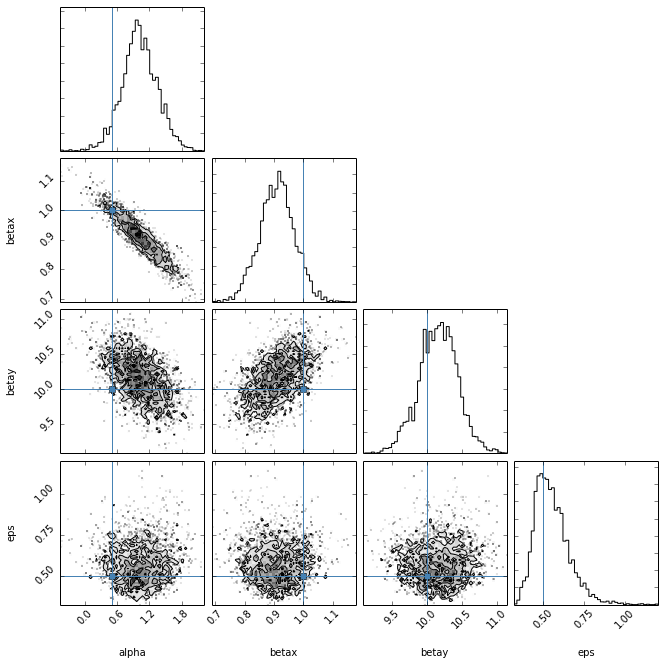PyMC Example¶

pymc is a python module that implements several MCMC sampling algorithms. Currently, pymc's stable release (2.x) mostly relised on the Gibbs and Metropolis-Hastings samplers, which are not that exciting, but the development version (3.x) has Hamiltonian Monte Carlo (HMC). pymc 2.x is available from github

https://github.com/pymc-devs/pymc/tree/2.3

You can also install via pip or easy_install. pymc requires a fortran compiler to build.

Similar to other examples, we start by making some fake data. In this case, we're going to do have a linear regression model with two predictor variables (x,y) and one outcome variable (z). The relation between them is: $Z_n = \alpha + \beta_x x_n + \beta_y y_n + \epsilon$ where $\epsilon$ is a Gaussian noise term.

In :
from numpy import *
Nobs = 20
x_true = random.uniform(0,10, size=Nobs)
y_true = random.uniform(-1,1, size=Nobs)
alpha_true = 0.5
beta_x_true = 1.0
beta_y_true = 10.0
eps_true = 0.5
z_true = alpha_true + beta_x_true*x_true + beta_y_true*y_true
z_obs = z_true + random.normal(0, eps_true, size=Nobs)

We can plot the data through two diferent slices of the data. We'll color code each point by it's value of the other predictor to see the trend in both cases.
In :
%matplotlib inline
from matplotlib import pyplot as plt
plt.figure(figsize=(12,6))
plt.subplot(1,2,1)
plt.scatter(x_true, z_obs, c=y_true, marker='o')
plt.colorbar()
plt.xlabel('X')
plt.ylabel('Z')
plt.subplot(1,2,2)
plt.scatter(y_true, z_obs, c=x_true, marker='o')
plt.colorbar()
plt.xlabel('Y')
plt.ylabel('Z')

Out:
<matplotlib.text.Text at 0x10e9ab0d0>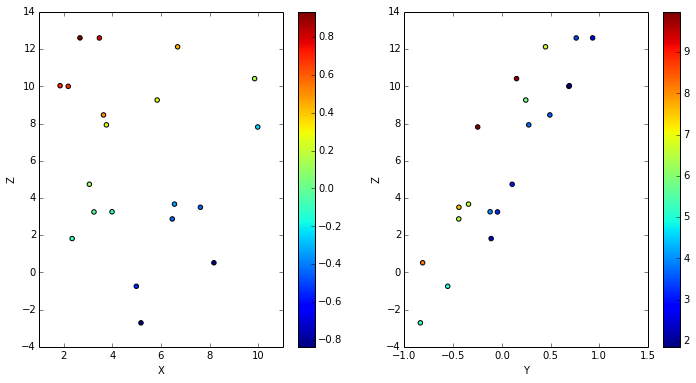Similar to PyStan, we build a model in a hiarchical manner, but instead of writing it out in another language, we use nothing but python code.

In :
import pymc
# define the parameters with their associated priors
alpha = pymc.Uniform('alpha', -100,100, value=median(z_obs))
betax = pymc.Uniform('betax', -100,100, value=std(z_obs)/std(x_true))
betay = pymc.Uniform('betay', -100,100, value=std(z_obs)/std(y_true))
eps = pymc.Uniform('eps', 0, 100, value=0.01)

# Now define the model
@pymc.deterministic
def model(alpha=alpha, betax=betax, betay=betay, x=x_true, y=y_true):
return alpha + betax*x + betay*y

# pymc parametrizes the width of the normal distribution by tau=1/sigma**2
@pymc.deterministic
def tau(eps=eps):
return power(eps, -2)

# Lastly relate the model/parameters to the data
data = pymc.Normal('data', mu=model, tau=tau, value=z_obs, observed=True)


The pymc model is defined solely in terms of python objects, referred to as stochastics (in the Bayesian sense, they are random variables). The four parameters are given uniform priors (other, more complicated priors could be used).

We then define the model as a python function with a special decorator: pymc.deterministic. This tells pymc that model is a function that depends on stochastic objects, but is itself a deterministic function of them (it's a function of random variables, not a random variable itself). The same is true for the function tau that simply converts the standard deviation parameter into a precision parameter. The last statement then tells pymc that the observed data z_obs is drawn from a normal distribution with mean equal to the model, presicsion equal to $1/\epsilon ^2$ and value equal to the observed data. The special observed=True argument tells pymc that this stochastic's value should remain constant.

With all the definitions in place, we simply feed the pymc objects into a pymc.MCMC to create a sampler and run it.

In :
sampler = pymc.MCMC([alpha,betax,betay,eps,model,tau,z_obs,x_true,y_true])
scales={alpha:0.1, betax:0.1, betay:1.0, eps:0.1})
sampler.sample(iter=10000)

[****************100%******************]  10000 of 10000 complete


Before running the sampler, I use the use_step_method to choose the adaptive version of the Metropolis-Hastings sampler. This is a more intelligent sampler than the default Metropolis-Hastings. It uses previous samples in the chain to construct a covariance matrix to more efficiently sample the parameter space. But it can be very finicky and you have to pick your initial scales carefully. We can plot the traces to see who things have gone:
In :
pymc.Matplot.plot(sampler)

Plotting alpha_0
Plotting betax_0
Plotting betay_0
Plotting tau_0
Plotting eps_0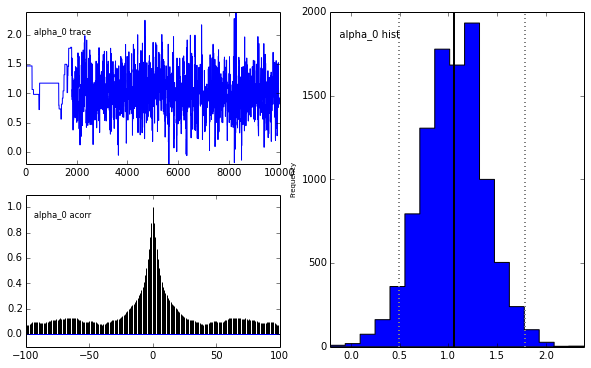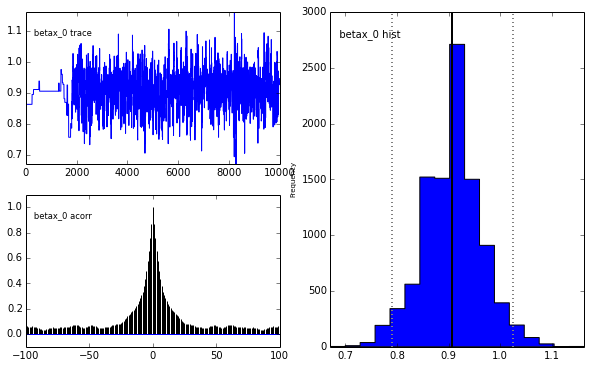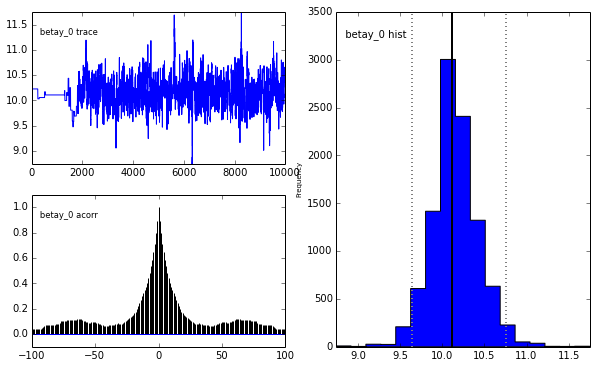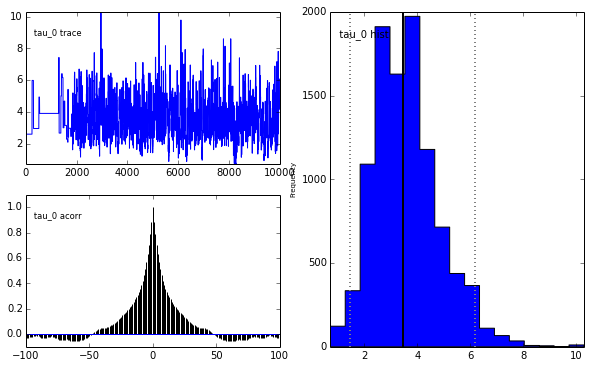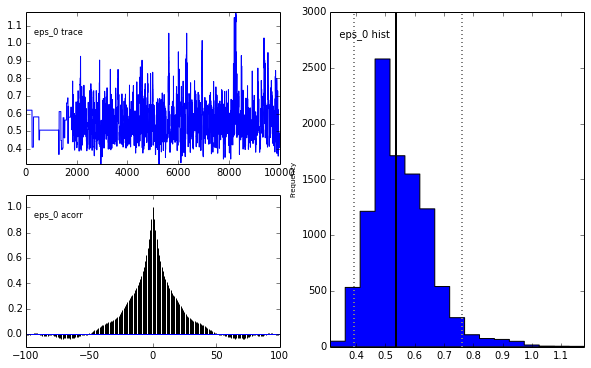For each stochastic, pymc plots the trace (upper-left panel), the auto-correlation (lower-left pane), and the histogram of the samples (right panel).

You'll see that nothing much happens at first, then the adaptive MH sampler finds a better covariance matrix and it starts to sample more efficiently. You can either throw up the first iterations, or just go for another sampling now that we're confident the sampler is behaving appropriately.

In :
sampler.sample(iter=10000)

[****************100%******************]  10000 of 10000 complete



Now we can do the statistics to find the solution. We can print out some summary statistics using pymc's internals.

In :
alpha.summary()


alpha:

Mean             SD               MC Error        95% HPD interval
------------------------------------------------------------------
1.034            0.329            0.011            [ 0.356  1.669]

Posterior quantiles:

2.5             25              50              75             97.5
|---------------|===============|===============|---------------|
0.37             0.818           1.031          1.233         1.692



And of course, you can always plot the results to see how they look. But this time, we can correct for the other predictor's effect. First, let's get the median of each variable from the samples. We get at the MCMC samples by looking at each variable's trace.

In :
m_alpha = median(alpha.trace())
m_betax = median(betax.trace())
m_betay = median(betay.trace())
m_eps = median(eps.trace())


The median is more robust toward non-symmetric distributions (such as the one for eps). Now we can plot the results, this time correcting for the y predictor when plotting versus x and vice versa. That way we can make sure the correct trend has been found. We can also plot a shaded region denoting the intrinsic dispersion.

In :
plt.figure(figsize=(12,6))
plt.subplot(1,2,1)
plt.plot(x_true, z_obs-m_alpha-m_betay*y_true, 'o')
plt.xlabel('X')
plt.ylabel('Z - alpha - beta_y y')
# Now plot the model
xx = array([x_true.min(), x_true.max()])
plt.plot(xx, xx*m_betax)
plt.plot(xx, xx*m_betax + m_eps, '--', color='k')
plt.plot(xx, xx*m_betax - m_eps, '--', color='k')
plt.subplot(1,2,2)
plt.plot(y_true, z_obs-m_alpha-m_betax*x_true, 'o')
plt.xlabel('Y')
plt.ylabel('Z - alpha - beta_x x')
yy = array([y_true.min(), y_true.max()])
plt.plot(yy, yy*m_betay)
plt.plot(yy, yy*m_betay + m_eps, '--', color='k')
plt.plot(yy, yy*m_betay - m_eps, '--', color='k')

Out:
[<matplotlib.lines.Line2D at 0x10d37fc50>]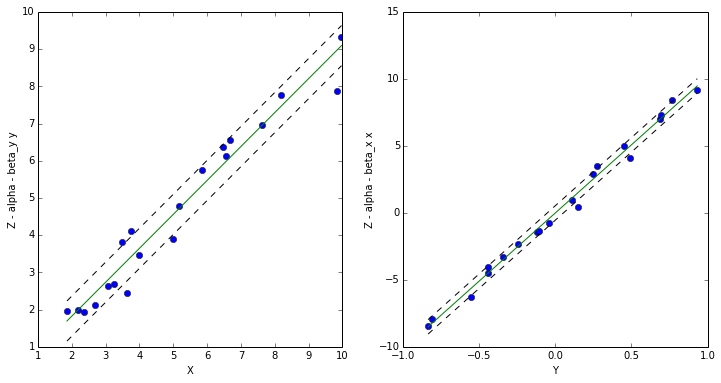If you have the triangle_plot plotting package, it's an easy way to look at the covariance of the various parameters. For this to work, we need the Markov chains in a 2D array indexed by [i,p], so we need to reshape the array to combine the 4 chains. pystan also includes a 5th "parameter", which is the log-probability at each step, so we can leave that out.

In :
samples = array([alpha.trace(),betax.trace(),betay.trace(),eps.trace()]).T
samples = samples
import triangle
tmp = triangle.corner(samples[:,:], labels=['alpha','betax','betay','eps'],
truths=[alpha_true, beta_x_true, beta_y_true, eps_true])